Next: 2.3.3.3 Linear Capacitor Up: 2.3.3 Devices Previous: 2.3.3.1 Conductor

### 2.3.3.2 Resistor

The constitutive relations for a linear temperature dependent resistor are

 V = I . R(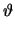) (2.32) R() = R0 .1 +. (-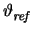)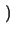(2.33) P = V . I (2.34)

The same considerations as for conductors apply for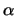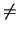0 . The stamp is given as
 yx, y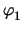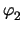I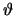f n1 1 - I n2 -1 I I -1 1 R R0 .. I V - I . R- I I - V P
As the node voltages and the branch current are independent solution variables, it is not guaranteed that the expression V - I . R equals zero while iterating towards the final solution. In the above stamp, the independent solution variable for the current I can be eliminated making I a dependent variable. The resulting stamp reads
 yx, yf n1 G - G V .. R0 . G2 - I n2 - G G - V .. R0 . G2 I-2 . V . G 2 . V . G I2 .. R0 P
with G = 1/R. Since I is a dependent variable, I and V may be used interchangeably, as long as R0. The above stamp is, of course, equal to the result obtained by directly considering the conductor G = 1/R which, in this case, depends in a non-linear way on. However, the current I can only be eliminated if R0 so that the second stamp is somewhat more restrictive. Furthermore, this procedure shows how additional currents can be added or eliminated whenever needed as long as a unique inversion V = g-1(I) of the the branch relation I = g(V) exists which is not the case for R = 0. Of course, I must not be used by other device models and hence be a local quantity of the device. In addition, V and I need not necessarily be defined for the same branch as is the case for current-controlled voltage sources.

Another interesting application can be found when adding the branch current for a conductor as an unknown. Neglecting temperature dependencies, the stamp reads

 yx, yI f n1 1 - I n2 -1 I I - G G 1 V . G - I
For an open circuit G = 0 while R = 0 results in a short circuit. By combining the above stamp with the stamp of the ideal resistor an ideal switch can be implemented whose stamp reads
 yx, yI f n1 1 - I n2 -1 I I - S S 1 - S V . S - I . (1 - S)
with S denoting the state of the switch. S = 1 gives a short circuit while S = 0 results in an open circuit.Next: 2.3.3.3 Linear Capacitor Up: 2.3.3 Devices Previous: 2.3.3.1 Conductor
Tibor Grasser
1999-05-31